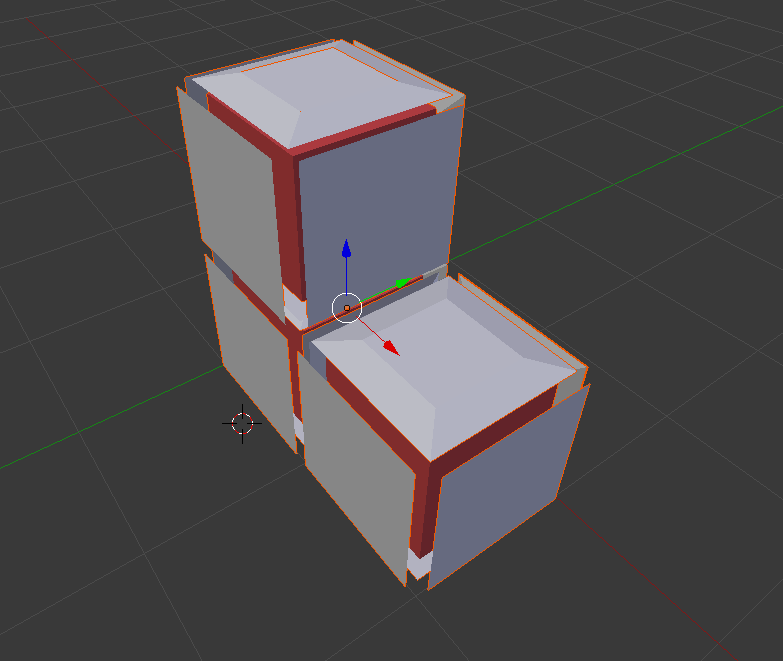DGtal  1.3.beta
io/boards/dgtalBoard3D-2-ks.cpp

Example of Khalimsky Signed Cell export with Display3D.

Export objects with Board3DIllustration of the resulting exported file (OBJ format visualized with blender).
#include <iostream>
#include "DGtal/io/boards/Board3D.h"
#include "DGtal/base/Common.h"
#include "DGtal/helpers/StdDefs.h"
#include "DGtal/shapes/Shapes.h"
#include "DGtal/io/DrawWithDisplay3DModifier.h"
using namespace std;
using namespace DGtal;
using namespace Z3i;
// Standard services - public :
int main( )
{
Point plow(0,0,0);
Point pup(1,1,0);
Domain domain( plow, pup );
K.init( plow, pup, true );
// drawing cells of dimension 3
SCell v2 = K.sSpel( Point( 1, 0, 0 ), KSpace::POS ); // +v
SCell v3 = K.sSpel( Point( 0, 1, 0 ), KSpace::POS ); // +v
SCell v4 = K.sSpel( Point( 1, 1, 0 ), KSpace::NEG ); // +v
SCell v = K.sSpel( Point( 0, 0, 0 ), KSpace::POS ); // +v
board << SetMode3D( v.className(), "Illustration" );
board << v << v2 << v3;
board.saveOBJ("board3D-2-ks.obj");
// Surfel of Voxel (0,0)
SCell sx = K.sIncident( v, 0, true ); // surfel further along x
SCell sy = K.sIncident( v, 1, true ); // surfel further along y
SCell sz = K.sIncident( v, 2, true ); // surfel further along z
SCell sxn = K.sIncident( v, 0, false ); // surfel further along x
SCell syn = K.sIncident( v, 1, false ); // surfel further along y
SCell szn = K.sIncident( v, 2, false ); // surfel further along z
// Resizing and shifting the surfel towords its associated voxel (v).
DGtal::TransformedPrism tsxn (sxn, v);
DGtal::TransformedPrism tsyn (syn, v);
DGtal::TransformedPrism tszn (szn, v);
board2 << tsx << tsy << tsz << tsxn << tsyn << tszn;
// Surfel of Voxel (1,0)
SCell sx2 = K.sIncident( v2, 0, true ); // surfel further along x
SCell sy2 = K.sIncident( v2, 1, true ); // surfel further along y
SCell sz2 = K.sIncident( v2, 2, true ); // surfel further along z
SCell sxn2 = K.sIncident( v2, 0, false ); // surfel further along x
SCell syn2 = K.sIncident( v2, 1, false ); // surfel further along y
SCell szn2 = K.sIncident( v2, 2, false ); // surfel further along z
// Resizing and shifting the surfel towords its associated voxel (v2).
DGtal::TransformedPrism tsx2 (sx2, v2);
DGtal::TransformedPrism tsy2 (sy2, v2);
DGtal::TransformedPrism tsz2 (sz2, v2);
DGtal::TransformedPrism tsxn2 (sxn2, v2);
DGtal::TransformedPrism tsyn2 (syn2, v2);
DGtal::TransformedPrism tszn2 (szn2, v2);
board2 << tsx2 << tsy2 << tsz2 << tsxn2 << tsyn2 << tszn2;
// Surfel of Voxel (0,1)
SCell sx3 = K.sIncident( v3, 0, true ); // surfel further along x
SCell sy3 = K.sIncident( v3, 1, true ); // surfel further along y
SCell sz3 = K.sIncident( v3, 2, true ); // surfel further along z
SCell sxn3 = K.sIncident( v3, 0, false ); // surfel further along x
SCell syn3 = K.sIncident( v3, 1, false ); // surfel further along y
SCell szn3 = K.sIncident( v3, 2, false ); // surfel further along z
// Shifting the surfel to its associated voxel (v3).
DGtal::TransformedPrism tsx3 (sx3, v3);
DGtal::TransformedPrism tsy3 (sy3, v3);
DGtal::TransformedPrism tsz3 (sz3, v3);
DGtal::TransformedPrism tsxn3 (sxn3, v3);
DGtal::TransformedPrism tsyn3 (syn3, v3);
DGtal::TransformedPrism tszn3 (szn3, v3);
board2 << tsx3 << tsy3 << tsz3 << tsxn3 << tsyn3 << tszn3;
std::cout << "save obj" << std::endl;
board2.saveOBJ("board3D-2bis-ks.obj");
}
DGtal::TransformedPrism
class to modify the position and scale to construct better illustration mode.
Definition: DrawWithDisplay3DModifier.h:179
DGtal::HyperRectDomain< Space >
DGtal::KhalimskySpaceND::init
bool init(const Point &lower, const Point &upper, bool isClosed)
Specifies the upper and lower bounds for the maximal cells in this space.
K
KSpace K
Definition: testCubicalComplex.cpp:62
DGtal::Board3D
The class Board3D is a type of Display3D which export the figures in the format OBJ/MTL when calling ...
Definition: Board3D.h:81
DGtal::KhalimskySpaceND::sSpel
SCell sSpel(Point p, Sign sign=POS) const
From the digital coordinates of a point in Zn, builds the corresponding spel (cell of maximal dimensi...
DGtal::SignedKhalimskyCell
Represents a signed cell in a cellular grid space by its Khalimsky coordinates and a boolean value.
Definition: KhalimskySpaceND.h:208
DGtal::Board3D::saveOBJ
void saveOBJ(const std::string &filename, const bool isNormalized=false)
DGtal
DGtal is the top-level namespace which contains all DGtal functions and types.
DGtal::KhalimskySpaceND::POS
static const constexpr Sign POS
Definition: KhalimskySpaceND.h:432
DGtal::SetMode3D
Modifier class in a Display3D stream. Useful to choose your own mode for a given class....
Definition: DrawWithDisplay3DModifier.h:73
main
int main(int argc, char **argv)
Definition: testArithmeticDSS-benchmark.cpp:147
domain
Domain domain
Definition: testProjection.cpp:88
DGtal::KhalimskySpaceND::sIncident
SCell sIncident(const SCell &c, Dimension k, bool up) const
Return the forward or backward signed cell incident to [c] along axis [k], depending on [up].
Point
MyPointD Point
Definition: testClone2.cpp:383
DGtal::KhalimskySpaceND::NEG
static const constexpr Sign NEG
Definition: KhalimskySpaceND.h:433
DGtal::KhalimskySpaceND
Aim: This class is a model of CCellularGridSpaceND. It represents the cubical grid as a cell complex,...
Definition: KhalimskySpaceND.h:64